Click to Chat

1800-1023-196

+91-120-4616500

CART 0

• 0

MY CART (5)

Use Coupon: CART20 and get 20% off on all online Study Material

ITEM
DETAILS
MRP
DISCOUNT
FINAL PRICE
Total Price: Rs.

There are no items in this cart.
Continue ShoppingArrhenius Theory of Electrolytic Dissociation

Table of Content

The Main Points of the Theory are

Evidences in Favour of Ionic Theory

Ions Present in Solid Electrolytes

Ohm's Law Applicability

Ionic Reaction

Heat of Neutralization

Abnormal Colligative Properties

Colour of Solution

Explanation of Some Other Phenomena

Limitations of Arrhenius Theory

Factors Pertaining to Degree of Ionization

Nature of Solute

Nature of Solvent

Dilution

Temperature

Related ResourcesIn order to explain the properties of electrolytic solutions, Arrhenius put forth, in 1884, a comprehensive theory which is known as theory of electrolytic dissociation or ionic theory.

The Main Points of the Theory are

An electrolyte, when dissolved in water, breaks up into two types of charged particles, one carrying a positive charge and the other a negative charge. These charged particles are called ions. Positively charged ions are termed cations and negatively charged as anions.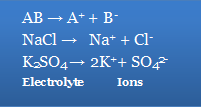In its modern form, the theory assumes that solid electrolytes are composed of ions which are held together by electrostatic forces of attraction. When an electrolyte is dissolved in a solvent, these forces are weakened and the electrolyte undergoes dissociation into ions. The ions are solvated.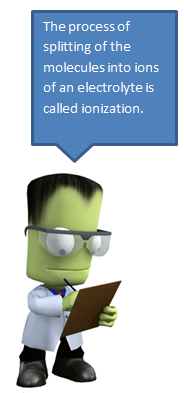The process of splitting of the molecules into ions of an electrolyte is called ionization. The fraction of the total number of molecules present in solution as ions is known as degree of ionization or degree of dissociation. It is denoted by

It has been observed that all electrolytes do not ionize to the same extent.
Some are almost completely ionized while others are feebly ionized. The degree of ionization depends on a number of factors.

Ions present in solution constantly re-unite to form neutral molecules and, thus, there is a state of dynamic equilibrium between the ionized the ionized and non-ionised molecules, i.e., AB   A+ + B-
Applying the law of mass action to above equilibrium [A+ ][B- ] /[AB] =K
K is known as ionization constant. The electrolytes having high  value of K are termed strong electrolytes and those having low value of K as weak electrolytes.

When an electric current is passed through the electrolytic solution, the positive ions (cations) move towards cathode and the negative ions (anions) move towards anode and get discharged, i.e., electrolysis occurs.
The ions are discharged always in equivalent amounts, no matter what their relative speeds are.

The electrolytic solutions is always neutral in nature as the total charge on one set of ions is always equal to the total charge on the other set of ions. However, it is not necessary that the number of two sets of ions must be equal always.

AB   A+ + B-

NaCl   Na+ + Cl-

(Both ions are equal)

AB2   A2+ + 2B-

BaCl2   Ba2+ + 2Cl-

(Anions are double that of cations)

A2B  2a+ + B2-

Na2SO4  2Na+ +

(Anions are double that of cations)

The properties of electrolytes in solution are the properties of ions present in solution. For example, acidic solution always contains H+ ions while basic solution contains OH- ions and characteristic properties of solutions are those of H- ions and OH- ions respectively.

The ions act like molecules towards depressing the freezing point, elevating the boiling point, lowering the vapour pressure and establishing the osmotic pressure.

The conductively of the electrolytic solution depends on the nature and number of ions as the current is carried through solution by the movement of ions.

Evidences in Favour of Ionic Theory

A large number of experimental observations are available which support Arrhenius theory. A few of them are given below:

Ions Present in Solid Electrolytes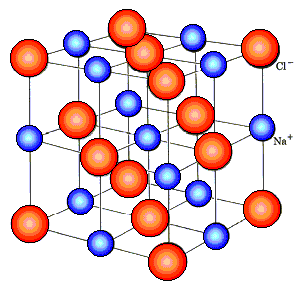X-ray diffraction studies have shown that electrolytes are composed of ions. For example, a crystal of NaCl does not contain NaCl units but Na+ and Cl- ions. Each Na+ ion is surrounded by six Cl- ions and each Cl- ion in turn is surrounded by six Na+ and Cl- ions. The ionic compounds behave as good conductors in fused state. It can only be possible of ions are already present in ionic solids.

Ohm's Law Applicability

The electrolytic solutions like metallic conductors obey Ohm's law, i.e., the strength of the current flowing conductor is directly proportional to potential difference (E) applied across the conductor and is inversely proportional to the resistance of the conductor. Mathematically, I = E/R

This can only be possible if ions are already present in the solution and no part of the current has only directive effect on the ions.Ionic Reaction

Evidence for the existence of ions in aqueous solutions of electrolytes is furnished by well known reactions in inorganic chemistry. A white precipitate of silver chloride is obtained whenever Ag+ ions come in contact with chloride ions.

Ag+  + Na+ Cl-  → AgCl + Na+ + NO3-

But no precipitation occurs when AgNO3 solution is added to CCl4, CHCl3 or C2H5Cl as these substances being non-electrolytes do not furnish Cl- ions in solution.

An acid which gives all tests of H+ ions in aqueous solution, does not give the same tests when dissolved in any organic solvent because no ionization of the acid occurs in the common reaction.

Heat of Neutralization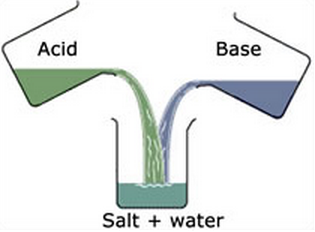When one gram equivalent of a strong acid is neutralized by one gram equivalent of a strong base, the heat evolved is always the same, i.e., 13.7 kcal. This can be explained on the basis of Arrhenius theory that an acid furnished H+ ions and base OH- ions when dissolved in water and the process of neutralization involves the common reaction.

H+ + OH-  H2O + 13.7 kcal.

Thus, heat of neutralization is actually the heat of formation of H2O from H+ and OH- ions.

Abnormal Colligative Properties

The abnormal behavior towards colligative properties as observed in the case of electrolytes can be explained on the basis of ionic theory. When an electrolyte is dissolved in water, the number of molecules actually dissolved due to ionization. The can't Hoff factor,

is always more than one, i.e., i = 1 + (n-1)  where 'n' is the number of ions produced by the ionization of one molecule of the electrolyte and ' is the degree of ionization.

Colour of Solution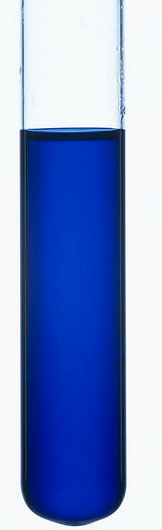The color of the electrolytes in solution. If any, is due to their ions, the CuSO4 is blue in solution due to the presence of Cu2+ ions. Potassium permanganate (KMnO4) is purple in solution due to the presence of  ions.

Explanation of Some Other Phenomena

Ionic theory provides satisfactory explanations regarding various phenomena such as electrolysis, conductivity, salt hydrolysis, solubility product, etc.

Limitations of Arrhenius Theory

Ostwald's dilution law which is based on Arrhenius theory is not applicable to strong electrolytes.

Strong electrolytes conduct electricity infused state, i.e., in absence of water. this is in contradiction of Arrhenius theory according to which the presence of solvent is a must for ionization.

Arrhenius theory assumes independent existence of ions but fails to account for the factors which influence the mobility of the ions.

Factors Pertaining to Degree of Ionization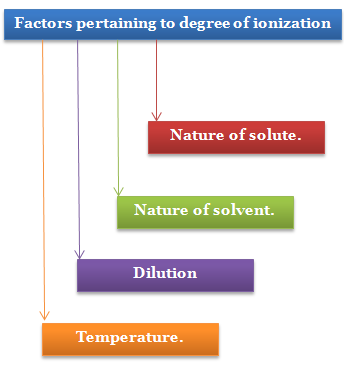The degree of ionization of an electrolyte in solution depends upon the following factors:

Nature of Solute

When the ionisable parts of a molecule of a substance are held more by covalent bonding than by electrovalent bonding, less ions are furnished in solution. Such substances are termed weak electrolytes. H2S, HCN, NH4OH, CH3COOH are examples of this class. NaCl, Ba(NO3)2, KOH, etc., are strong electrolytes, in which the transfer of electrons seems to be more or less complete, furnish ions immediately when dissolved. Strong electrolytes are almost completely ionized in solution.

Nature of Solvent

The main function of the solvent is to weaken the electrostatic forces of attraction between the two ions and separate them. The force of attraction holding the ions together in any medium is expressed as

F= 1/(K ) (q1 q2)/r2

where K is the dielectric constant of medium.

Any solvent which has high value of dielectric constant has the capacity of separating ions. Water is considered to be the best solvent as it has the highest dielectric constant. The dielectric constants of some of the solvents are given below at 250 C.

Solvent

Dielectric Constant

Water

81

Methyl alcohol

35

Ethyl alcohol

27

Acetone

21

Dilution

The extent of ionization of an electrolyte is inversely proportional to the concentration of its solution. Thus, degree of ionization increases with the increase of dilution of the solution, i.e., decreasing the concentration of the solution.

Temperature

The degree of ionization increases with the increase of temperature. This is due to the fact that at higher temperature molecular speed is increased which overcomes the forces of attraction between the ions.Question 1: The process of splitting of the molecules into ions of an electrolyte is called..

a. ionization

b. electrolysis

c. solvation

d. protonation

Question 2: The color of the electrolytes in solution is due to is..

a. ions

b. solvation

c. electrolysis

d. dissolution

Question 3: Degree of ionization does not depend upon..

a. temperature

b. pressure

c. concentration

d.nature of solvent

Question 4: Colour of CuSO4 solution is

a. green

b. yellow

c. blue

d. redQ.1

Q.2

Q.3

Q.4

a

a

b

c

Related Resources

Look into the Papers of Previous Years for an idea about type of questions asked in IIT JEE.

You can get the knowledge of Useful Books of Chemistry

You can also refer to Electrolysis and Electrolytic Cell

To read more, Buy study materials of Electrochemistry comprising study notes, revision notes, video lectures, previous year solved questions etc. Also browse for more study materials on Chemistry here.### Course Features

• 731 Video Lectures
• Revision Notes
• Previous Year Papers
• Mind Map
• Study Planner
• NCERT Solutions
• Discussion Forum
• Test paper with Video Solution